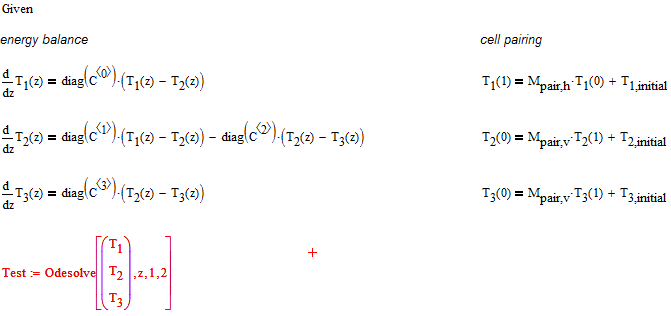cancel
Showing results for
Did you mean:
cancel
Showing results for
Did you mean:

## solving a system of n ODEs5-Regular Member

## solving a system of n ODEs

Dear all,

im trying to solve the energy balance equations of a system of heat exchangers that the in- and outlets are linked with the others. I think that the equation should look like this, but i cannot bring the Odesolve to function:Can someone tell me, how to finish that? Can Odesolve calculate those T.i(z) in vector? In the attached file, you can see my test value of pairing matrices and initial values.

Thx!21-Topaz II
(To:LertPianapitham)

Hi,

Consider the first differential equation in the solution block. In the cell-pairing column we have the product of the matrix M.pairh for a vector that  gives a vector, and to which is added the vector T1.initial, I suppose, therefore that T1 (z) is a vector !!! If on the right of an equation, there is a vector, also on the left there must be a vector and then T1 (z) is a vector as well as T2 (z) and T3 (z). And so it becomes all very strange. See below:Announcements
Top Tags